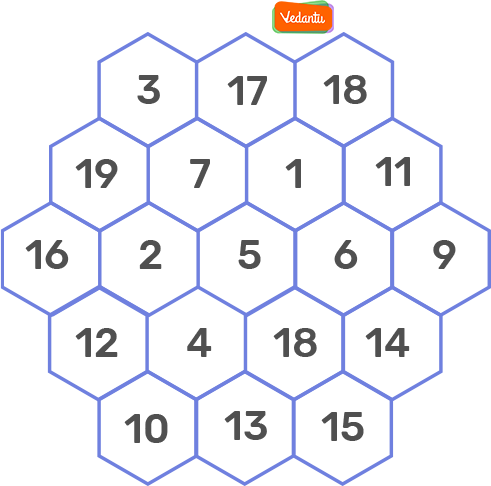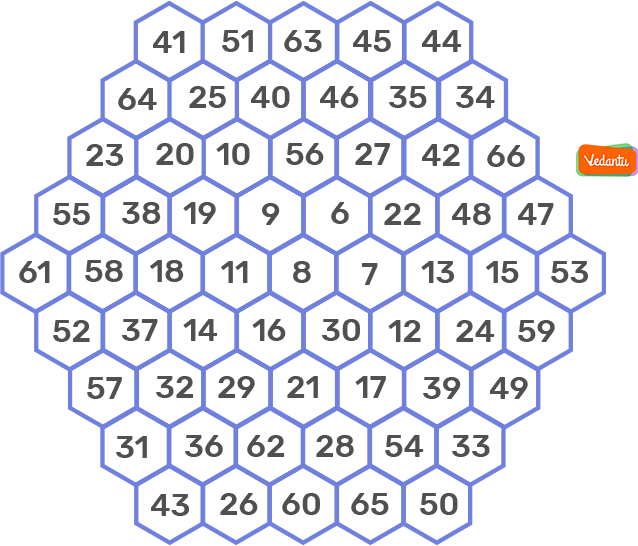Courses
Courses for Kids
Free study material
Free LIVE classes
More

# Magic Hexagon for Trig IdentitiesLIVE
Join Vedantu’s FREE Mastercalss

## Magic Hexagon

A magic hexagon for trigonometric identities of order ‘n’ is an arrangement of numbers in a centered hexagonal pattern having n cells on each edge, such that the numbers in each row, in all the three directions, sum up to the same magic constant. It appears that magic hexagons exist only for n = 1 (that is trivial) and n = 3. In addition, the solution of order 3 is typically unique.

### Normal Magical Hexagons

A normal magic hexagon consists of the consecutive integers from 1 to 3n² − 3n + 1, while an abnormal one starts with a number other than one. Opposed to trig magic hexagons, normal magical hexagons with order greater than 3 just do not exist, however certain abnormal ones do. As stated, abnormal magic hexagons means starting the sequence of numbers with something besides one.

Examples for n = 3, and 5 are showcased below.

n = 3 (Magic Constant = 38):n = 5 (Magic Constant = 244):### Trigonometry Hexagon

Using the hexagon drawing, you will create a memory trick to understand the product/quotient, reciprocal, Pythagorean. An identity is an equation which is true for all x-values.

### Building the Trig Hexagon Identities

In order to build the trig identities hexagon, you would require following the given steps:

1. Construct a hexagon and mark a “1” in the center.

2. Write ‘tan’ on the farthest of the left vertex.

3. Apply the Quotient Identity for tangent going clockwise.

4. Fill in the Reciprocal Identities on the opposite vertices.

### Pythagorean Identities

For each of the shaded triangles, the upper left function squared plus the upper right function squared is equivalent to the bottom function squared.

### Deriving the Pythagorean Identities

A is the center of the Unit Cirlcle.

B is a point on the circle in the 1st

C is at a right angle.

1. State the ratio BC/AB with respect to 𝑥. This is the length measurement of side a.

2. State the ratio AC/AB with respect to 𝑥. This is the length measurement of side b.

3. Substitute the expressions you discovered in #1 and #2 into the Pythagorean Theorem for the purpose of creating the first Pythagorean Identity.

4. Does the Pythagorean Identity hold true in the other 3 quadrants? Why or why not?

5. Divide each term in the Pythagorean Identity in #3 by cos2 𝑥 for the purpose of deriving another form of the identity.

6. Divide each term in the Pythagorean Identity in #3 by sin2 𝑥 for the purpose of deriving another form of the identity.

### Even and Odd Identities

Recall that functions are taken into consideration even if their graphs consist of y-axis symmetry or odd if their graphs consist of rotational symmetry about the origin. Algebraically, 𝑓(−𝑥) = −𝑓(𝑥) holds true for all odd functions while 𝑓(−𝑥) = 𝑓(𝑥) holds true for all even functions. Firstly, sketch each parent graph and find out if the function is even or odd. Then you will write the identities using the algebraic definitions.

### Solved Examples

Example:

Can you help Alex, prove the following identity with the help of the trig identities?

{sin³θ + cos³θ/sinθ + cosθ + sinθcosθ} = 1

Solution:

We make use of the following identity:

a³ + b³ = (a + b) (a² − ab + b²)

We apply the Pythagorean identities in order to prove this identity.

LHS = {sin³θ + cos³θ/sinθ + cosθ + sinθcosθ}

= (sinθ + cosθ) (sin²θ - sinθ cosθ + cos²θ) / sinθ + cosθ + sinθ cosθ

= (sin²θ - sinθ cosθ + cos²θ) + sinθcosθ

= sin²θ + cos²θ

= 1

= RHS

Hence proved.

Last updated date: 18th Sep 2023
Total views: 281.7k
Views today: 7.81k

## FAQs on Magic Hexagon for Trig Identities

Q1. What are Trig Identities?

Answer: Trigonometric identities are mathematical equations that establish relation with different trigonometric functions and are true for any value of the variable that is present in the domain. Usually, an identity is an equation that holds true for all the values of the variable(s) that are there.

For example, some of the algebraic identities are as follows:

(a + b)² = a² + b² + 2ab

(a - b)² = a² - b² - 2ab

(a + b) (a - b)² = a² - b²

The algebraic identities relate only the variables. On the other hand, the trig identities relate the 6 trigonometric functions which essentially include sin, cos, tan, sec, cosec, and cot.

Q2. What is Meant by Cofunction Identities?

Answer: The trig functions such as cosine, cosecant and cotangent on the right side of the hexagon are the cofunctions of sine, secant and tangent on the left, respectively. Therefore, sine and cosine are the cofunctions; thus the “co” in cosine.

Cofunction identities are formed from x and π/2 − x being a pair of complementary angles. That implies they can be found going right to left or going left to right on the trigonometry hexagon.

Q3. What is Meant by Quotient Identities?

Answer: Along the external edges of the hexagon, any trig function is equivalent to the quotient of the functions on the following two consecutive vertices in either direction.